[Introduction]  [Signal arithmetic]  [Signals and noise]   [Smoothing]   [Differentiation]  [Peak Sharpening]  [Harmonic analysis]   [Fourier convolution]  [Fourier deconvolution]  [Fourier filter]  [Wavelets]   [Peak area measurement]  [Linear Least Squares]  [Multicomponent Spectroscopy]  [Iterative Curve Fitting]  [Hyperlinear quantitative absorption spectrophotometry] [Appendix and Case Studies]  [Peak Finding and Measurement]  [iPeak]   [iSignal]  [Peak Fitters]   [iFilter]  [iPower]  [List of downloadable software]  [Interactive tools]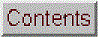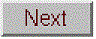## Signal arithmetic

[Subtraction]   [Division]   [Interpolation]  [Spreadsheets]   [Matlab]   [Octave]  [Getting data into Matlab/Octave]   [Spreadsheet or Matlab/Octave?]

The most basic signal processing functions are those that involve simple signal arithmetic: point-by-point addition, subtraction, multiplication, or division of two signals or of one signal and a constant. Despite their mathematical simplicity, these functions can be very useful. For example, in the left part of the figure below, the top curve is the optical absorption spectrum of an extract of a sample of oil shale, a kind of rock that is is a source of petroleum.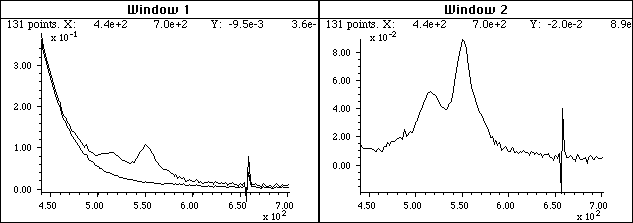A simple point-by-point subtraction of two signals allows the background (bottom curve on the left) to be subtracted from a complex sample (top curve on the left), resulting in a clearer picture of what is really in the sample (right). (X-axis = wavelength in nm; Y-axis = absorbance).

This optical spectrum exhibits two absorption peaks, at about 515 nm and 550 nm, that are due to a class of molecular fossils of chlorophyll called porphyrins. (Porphyrins are used as geomarkers in oil exploration). These absorption peaks are superimposed on a background absorption caused by the extracting solvents and by non-porphyrin compounds extracted from the shale. The bottom curve is the spectrum of an extract of a non-porphyrin-bearing shale, showing only the background absorption. To obtain the spectrum of the shale extract without the background, the background (bottom curve) is simply subtracted from the sample spectrum (top curve). The difference is shown in the right in Window 2 (note the change in Y-axis scale). In this case the removal of the background is not perfect, because the background spectrum is measured on a separate shale sample. However, it works well enough that the two bands are now seen more clearly and it is easier to measure precisely their absorbances and wavelengths. (Thanks to the late Prof. David Freeman for the spectra of oil shale extracts).

In this example and the one below, I am making the assumption that the two signals in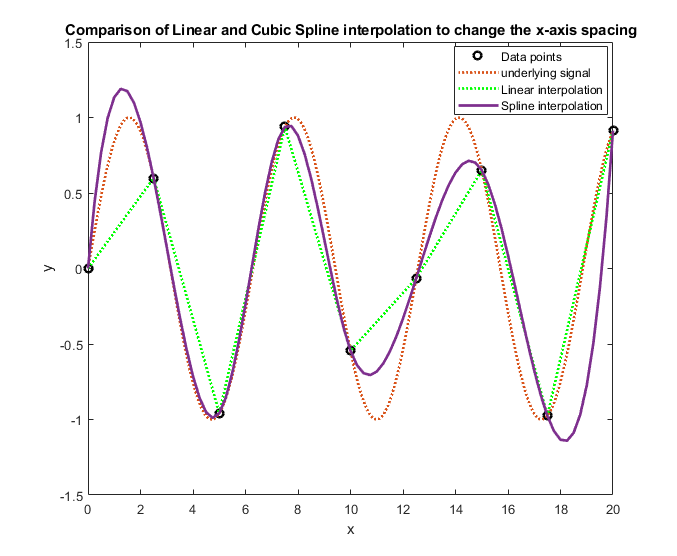Window 1 have the same x-axis values - in other words, that both spectra are digitized at the same set of wavelengths. Subtracting or dividing two spectra would not be valid if two spectra were digitized over different wavelength ranges or with different intervals between adjacent points. The x-axis values must match up point for point. In practice, this is very often the case with data sets acquired within one experiment on one instrument, but the experimenter must be careful if the instruments settings are changed or if data from two experiments or two different instrument are combined. It is possible to use the mathematical technique of interpolation to change the number of points or to equalize unequally-spaced x-axis intervals of signals; the results are only approximate but often close enough in practice. Matlab and Octave has several built in functions for linear and cubic spline interpolation; see the Matlab/Octave script CompareInterp1andSpline.m (graphic on the right) and CompareInterpolationMethods2.m (graphic). (Interpolation is one of the functions of my multi-purpose interactive iSignal function described later).

Sometimes one needs to know whether two signals have the same shape, for example in comparing the signal of an unknown to a stored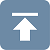reference signal. Most likely the amplitudes of the two signals, will be different. Therefore a direct overlay or subtraction of the two signals will not be useful. One possibility is to compute the point-by-point ratio of the two signals; if they have the same shape, the ratio will be a constant. For example, examine Figure 2.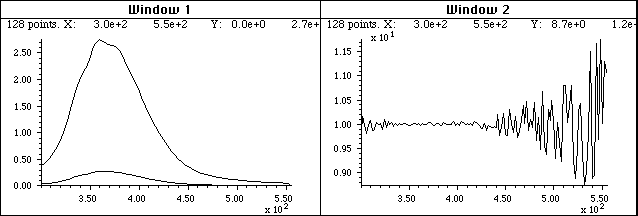Do the two signals on the left have the same shape? They certainly do not look the same, but that may simply be due to the fact that one is much weaker than the other. The ratio of the two signals, shown in the right part (Window 2), is relatively constant from 300 to 440 nm, with a value of 10 +/- 0.2. This means that the shape of these two signals is very nearly identical over this x-axis range.

The left part (Window 1) shows two superimposed signals, one of which is much weaker than the other. But do they have the same shape? The ratio of the two signals, shown in the right part (Window 2), is relatively constant from x=300 to 440, with a value of 10 +/- 0.2. This means that the shape of these two signals is the same, within about +/-2 %, over this x-axis range, and that top curve is very nearly 10 times more intense than the bottom one. Above x=440 the ratio is not even approximately constant; this is caused by noise, which is the subject of the next section.

A division by zero error will be caused by even a single zero in the denominator vector, but that can usually be avoided by applying a small amount of smoothing of the denominator, by adding a small positive number to the vector, or by using the custom Matlab/Octave function rmz.m (remove zeros), which replaces zeros with the nearest non-zero numbers, or the nlt.m (no lower than) function that limits the lowest number in a vector to a specified value.

On-line calculations and plotting. Wolfram Alpha is a Web site and a smartphone app that is a computational tool and information source, including capabilities for mathematics, plotting data and functions, vector and matrix manipulations, statistics and data analysis, and many other topics. Statpages.org can perform a huge range of statistical calculations and tests. There are several Web sites that specialize in plotting data, including Tableau, Plotly, Grapher, and Plotter. All of these require a reliable Internet connection, and they can be useful when you are working on a mobile device or a on computer that does not have the required software.

Popular spreadsheets such as Excel or Open Office Calc, have built-in functions for all common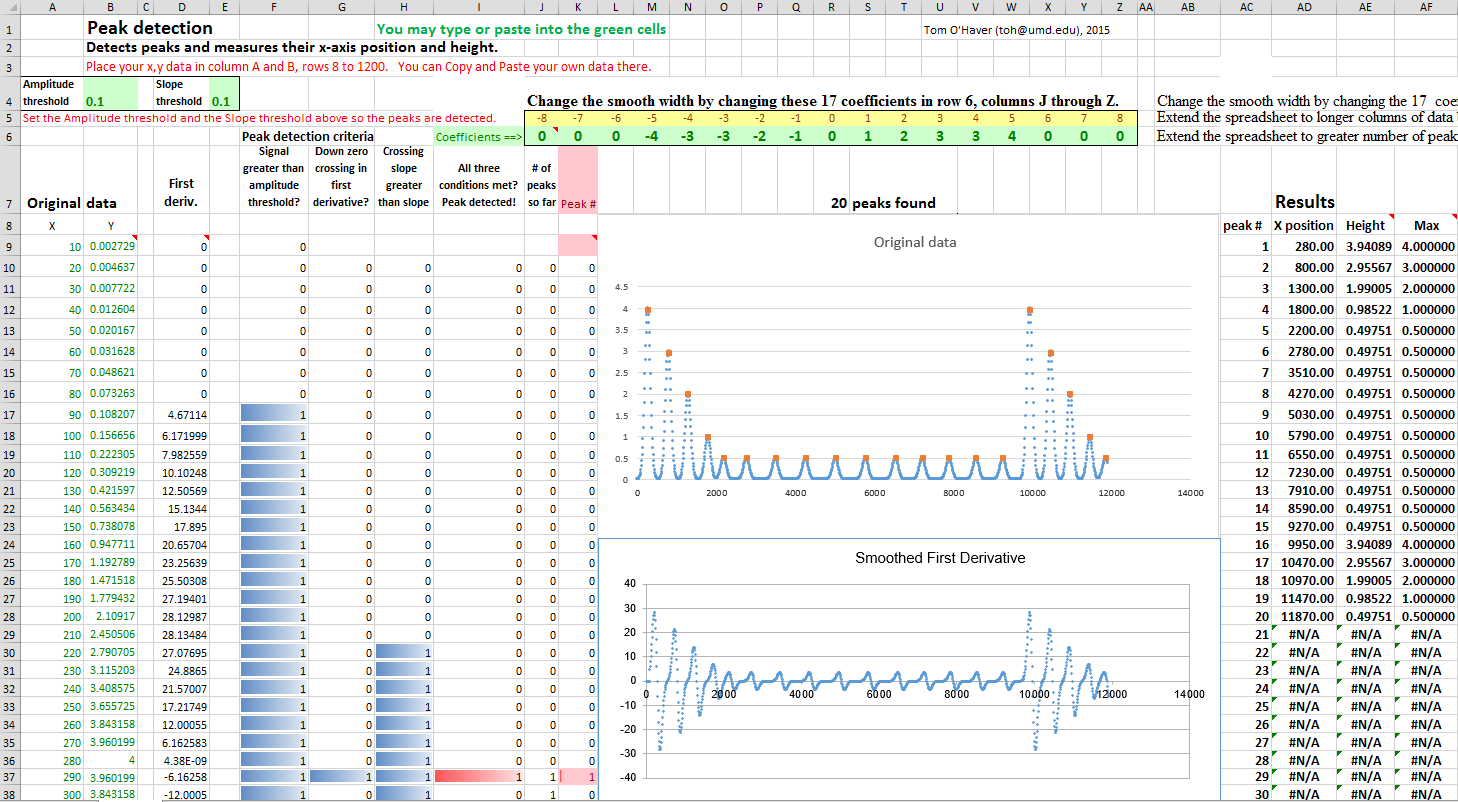math operations, named variables, x,y plotting, text formatting, matrix math, etc. (For a list of Excel functions with tutorials on how to use them, see https://computeexpert.com/english-blog/excel-formulas-list.) Spreadsheet cells can contain numerical values, text, mathematical expression, or references to other cells. A vector of values such as a spectrum can be represented as a row or column of cells; a rectangular array of values such as a set of spectra can be represented as a rectangular block of cells. User-created names can be assigned to individual cells or to ranges of cells, then referred to in mathematical expression by name. Mathematical expressions can be easily copied across a range of cells, with the cell references changing or not as desired. Plots of various types (including the all-important x-y or scatter graph) can be created by menu selection. See http://www.youtube.com/watch?v=nTlkkbQWpVk for a nice video demonstration. Both Excel and Calc offer a form design capability with full set of user interface objects such as buttons, menus, sliders, and text boxes; these can be user to create attractive graphical user interfaces for end-user applications, such as in http://terpconnect.umd.edu/~toh/models/. The latest versions of both Excel (Excel 2013) and OpenOffice Calc (3.4.1) can open and save either spreadsheet file format (.xls and .ods, respectively). Simple spreadsheets in either format are compatible with the other program. However, there are small differences in the way that certain functions are interpreted, and for that reason I supply most of my spreadsheets in .xls (for Excel) and in .ods (for Calc) formats.  See "Differences between the OpenDocument Spreadsheet (.ods) format and the Excel (.xlsx) format". Basically, Calc and do most everything Excel can do, but Calc is free to download and is more Windows-standard in terms of look-and-feel. Excel is more "Microsoft-y" and for some operations is faster than Calc. If you have access to Excel, I would use that.

If you are working on a tablet or smartphone, you could use the Excel mobile app, Numbers for iPad, or several other mobile spreadsheets. These can do basic tasks but do not have the fancier capabilities of the desktop computer versions. By saving their data in the "cloud" (e.g. iCloud or SkyDrive), these apps automatically sync changes in both directions between mobile devices and desktop computers, making them useful for field data entry.

Matlabis a "multi-paradigm numerical computing environment and fourth-generation programming language" (Wikipedia). In Matlab (and in Octave, its GNU clone), a single variable can represent either a single "scalar" value, a vector of values (such as a spectrum or a chromatogram), a matrix (a rectangular array of values, such as a set of spectra), or a set of multiple matrices. All the standard math operations and functions adjust to match. This greatly facilitates mathematical operations on signal waveforms.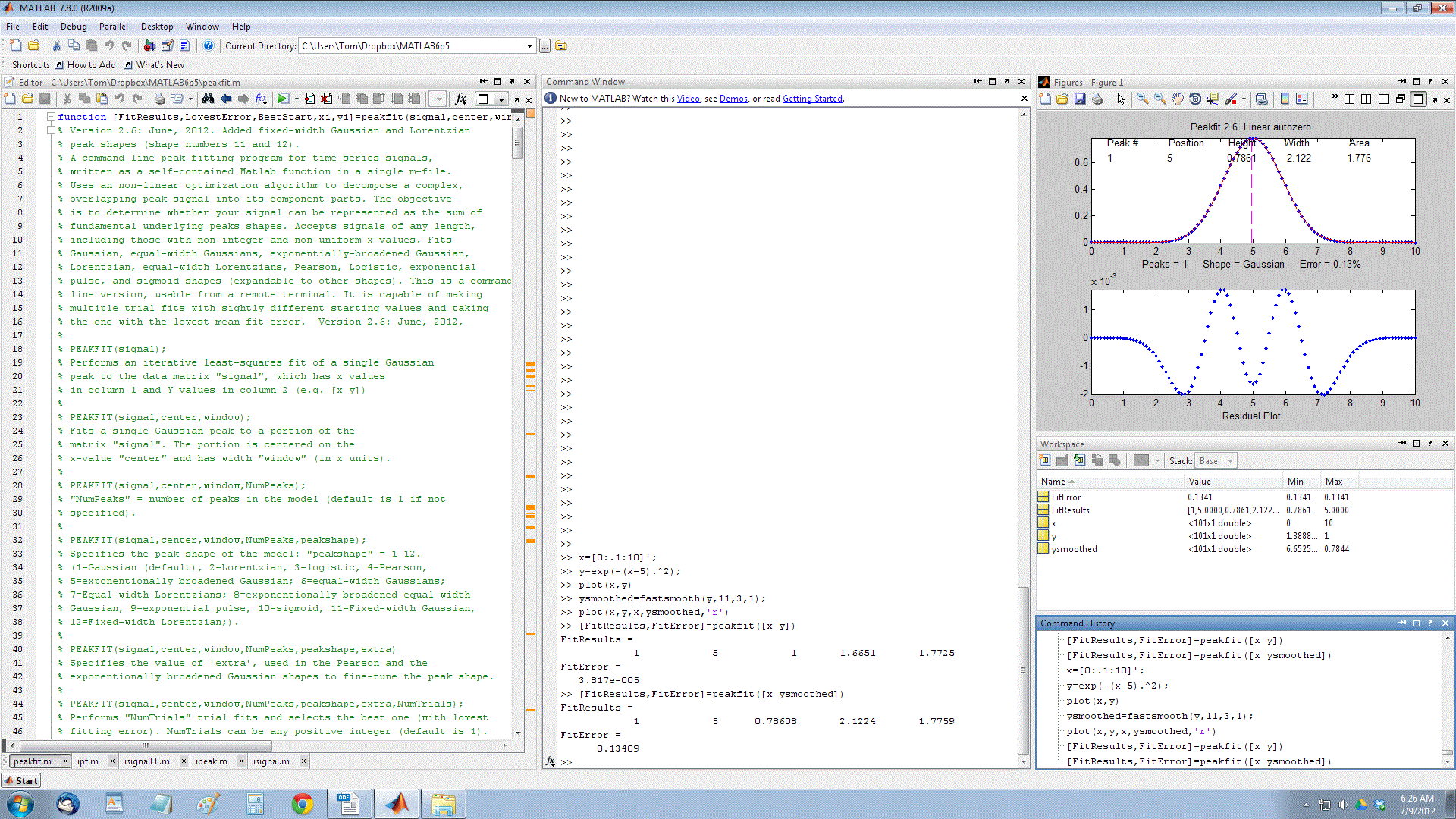For example, if you have signal amplitudes in the variable y, you can plot it just by typing "plot(y)". And if you also have a vector t of the same length containing the times at which each value of y was obtained, you can plot y vs t by typing "plot(t,y)". Two signals y and z can be plotted on the same time axis for comparison by typing "plot(t,y,t,z)" .  (Matlab automatically assigns different colors to each line, but you can control the color and line style yourself by adding additional symbols; for example "plot(y,y,'r.',y,z,'b-')" will plot y with red dots and z with a blue line.  You can divide up one figure window into multiple smaller plots by placing subplot(m,n,p) before the plot command to plot in the pth section of a m-by-n grid of plots. Here is a 2x2 example of a subplot. Type "help plot" or "help subplot" for more options. (Throughout this site, you can copy and paste, or drag and drop, any of the single-line or multi-line code examples into the Matlab or Octave editor or directly into the command line and press Enter to execute it immediately).

For publication-quality graphs, click on a Figure window, then click File > Export setup, choose the size, resolution, color, fonts, etc, then click Export and select the file format (e.g.TIF, eps, etc). You can also use PlotPub , a downloadable library that is free, easy to use, allows great flexibility in choosing graph details, and creates great-looking graphs within Matlab that can be exported in EPS, PDF, PNG and TIFF with adjustable resolution. Here's an example (script, graphic).

For real-time plotting in Matlab/Octave, see CaseStudies.html#realtime.

The function max(y) returns the maximum value of y and min(y) returns the minimum. Individual elements in a vector are referred to by index number; for example, t(10) is the 10th element in vector t, and t(10:20) is the vector of values of t from the 10th to the 20th entries. You can find the index number of the entry closest to a given value in a vector by using the downloadable val2ind.m function; for example,  t(val2ind(y,max(y))) returns the time of the maximum y, and  t(val2ind(t,550):val2ind(t,560)) is the vector of values of t between 550 and 560 (assuming t contains values within that range). The units of the time data in the t vector could be anything - microseconds, milliseconds, hours, any time units.

A Matlab variable can also be a matrix, a set of vectors of the same length combined into a rectangular array. For example, intensity readings of 10 different optical spectra, each taken at the same set of 100 wavelengths, could be combined into the 10x100 matrix S.  S(3,:) would be the third of those spectra and S(5,40) would be the intensity at the 40th wavelength of the 5th spectrum. The Matlab/ Octave scripts plotting.m and plotting2.m show how to plot multiple signals using matrices and subplots.

The subtraction of two signals a and b, as in Figure 1, can be performed simply by writing a-b. To plot the difference, you would write "plot(a-b)". Likewise, to plot the ratio of two signals, as in Figure 2, you would write "plot(a./b)". So, "./" means divide point-by-point and ".*" means multiply point-by-point. The * by itself means matrix multiplication, which you can use to perform repeated multiplications without using loops. For example, if x is a vector

A=[1:100]'*x;

creates a matrix A in which each column is x multiplied by the the numbers 1, 2,...100. It is equivalent to, but more compact and up to 300 times faster than, writing a "for"  loop like this:

for n=1:100;
A(:,n)=n.*x;
end;

Yes, that's right, it makes that much difference, at least in this simple example. It will be faster if you pre-allocate memory space for the A matrix by adding the statement A=zeros(100,100) before the loop. But even then the matrix notation is faster than the loop.

In Matlab/Octave, "/" is not the same as "\". Typing "b\a" will compute the "matrix right divide", in effect the weighted average ratio of the amplitudes of the two vectors (a type of least-squares best-fit solution), which in the example in Figure 2 will be a number close to 10. The point here is that Matlab doesn't require you to deal with vectors and matrices as collections of numbers; it knows when you are dealing with matrices, or when the result of a calculation will be a matrix, and it adjusts your calculations accordingly. See http://www.mathworks.com/help/matlab/matlab_prog/array-vs-matrix-operations.html.

Probably the most common errors you'll make in Matlab/Octave are punctuation errors, such as mixing up periods, commas, colons, and semicolons, or parentheses, square brackets, and curly brackets; type "help punct" at the Matlab prompt and read the help file until you fall asleep. Little things can mean a lot in Matlab. Another common error is getting the rows and columns of vectors and matrices mixed up. (Full disclosure: I still make all these kinds of mistakes all the time). Here's a text file that gives examples of common vector and matrix operations and errors in Matlab and Octave. If you are new to this, I recommend that you read this file and play around with the examples there. Writing Matlab is a trial and error process, with the emphasis on error.

There are many code examples in this text that you can Copy and Paste and modify into the Matlab/Octave command line, which is a great way to learn and is especially convenient if you can position the windows so that Matlab shares the screen with this website (e.g. Matlab on the left and web browser on the right (click for graphic) or (even better) if you have two monitors hooked to your computer configured to expand the desktop horizontally. If you try to run one of my scripts or functions and it gives you a "missing function" error, look for the missing item on functions.html, download it into your path, and try again.

Matlab Compiler is a separately available product that lets you share programs as standalone applications, and Matlab Compiler SDK lets you build C/C++ shared libraries, Microsoft .NET assemblies, Java classes, and Python packages from Matlab programs.

Getting data into Matlab/Octave.
You can easily import your own data into Matlab or Octave, for example by using the xlsread or importdata functions at the command line or in your own scripts. Data can be imported from plain text files (.txt), CSV files (comma separated values), from several image and sound formats, or from spreadsheets. For example, the following lines will read the first two columns of the csv file
"Sample_5.0ppm.csv" in the current folder and assign them to the vectors x and y.

x=mydata(:,1);
y=mydata(:,2);

The script "xlsreadDemo.m" provides a simple example of reading a multi-column spreadsheet “xlsx” file. For more complex spreadsheets, Matlab has a convenient Import Wizard (click File > Import Data) that gives you a preview into the data file, parses the data file looking for columns and rows of numeric data and their labels, and gives you a chance to select and re-label the desired variables and to choose the output type. You can even click on the little arrow to the right of "Import selection" and Matlab will write you a script that will perform those operations, which you can modify for other file types and formats.

JCAMP-DX is a standard file form for exchange of infrared spectra and related chemical and physical information between spectrometer data systems of different manufacture. Matlab’s jcampread function can import such data. For an example, see ReadJcampExample.m.

It is also possible to import data from graphical line plots or printed graphs by using the built-in "ginput" function that obtains numerical data from the coordinates of mouse clicks (as in DataTheif or Figure Digitizer).

Matlab R2013a or newer can even read the sensors on your iPhone or Android phone via Wi-Fi. To read the outputs of older analog instruments, you need an analog-to-digital converter, an Arduino microcontroller board, or a USB voltmeter.

To read the analog output signals of older analog instruments, you need an analog-to-digital converter, an Arduino microcontroller board, or a USB voltmeter. Mathworks has separate data acquisition toolbox for Matlab.

Python can import data in text, CSV, JSON, Matlab, and several other formats, using the Variable Explorer panel in the Spyder desktop, or through the separately downloadable Pandas Data Analysis package.

Matlab Versions.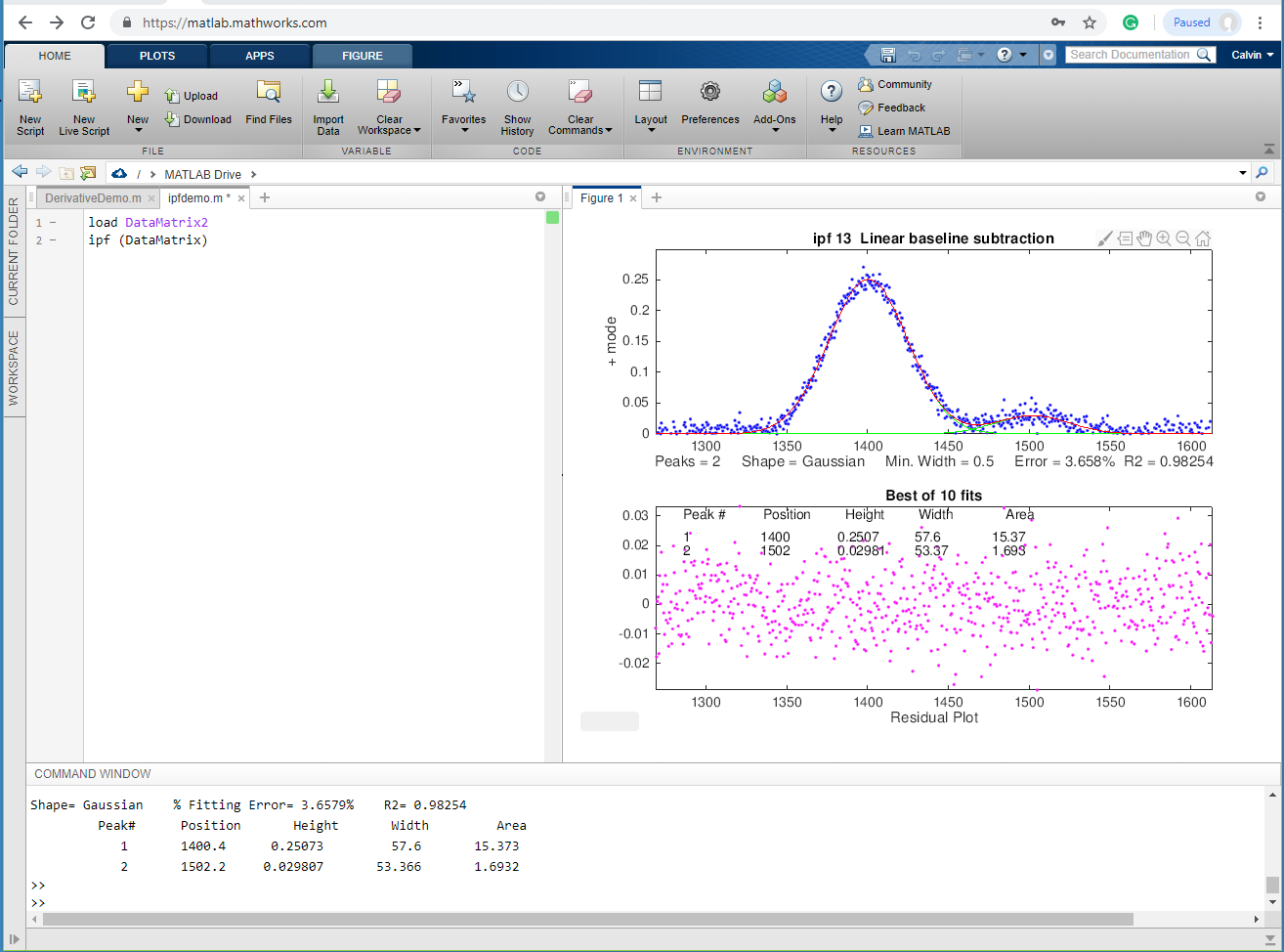The standard commercial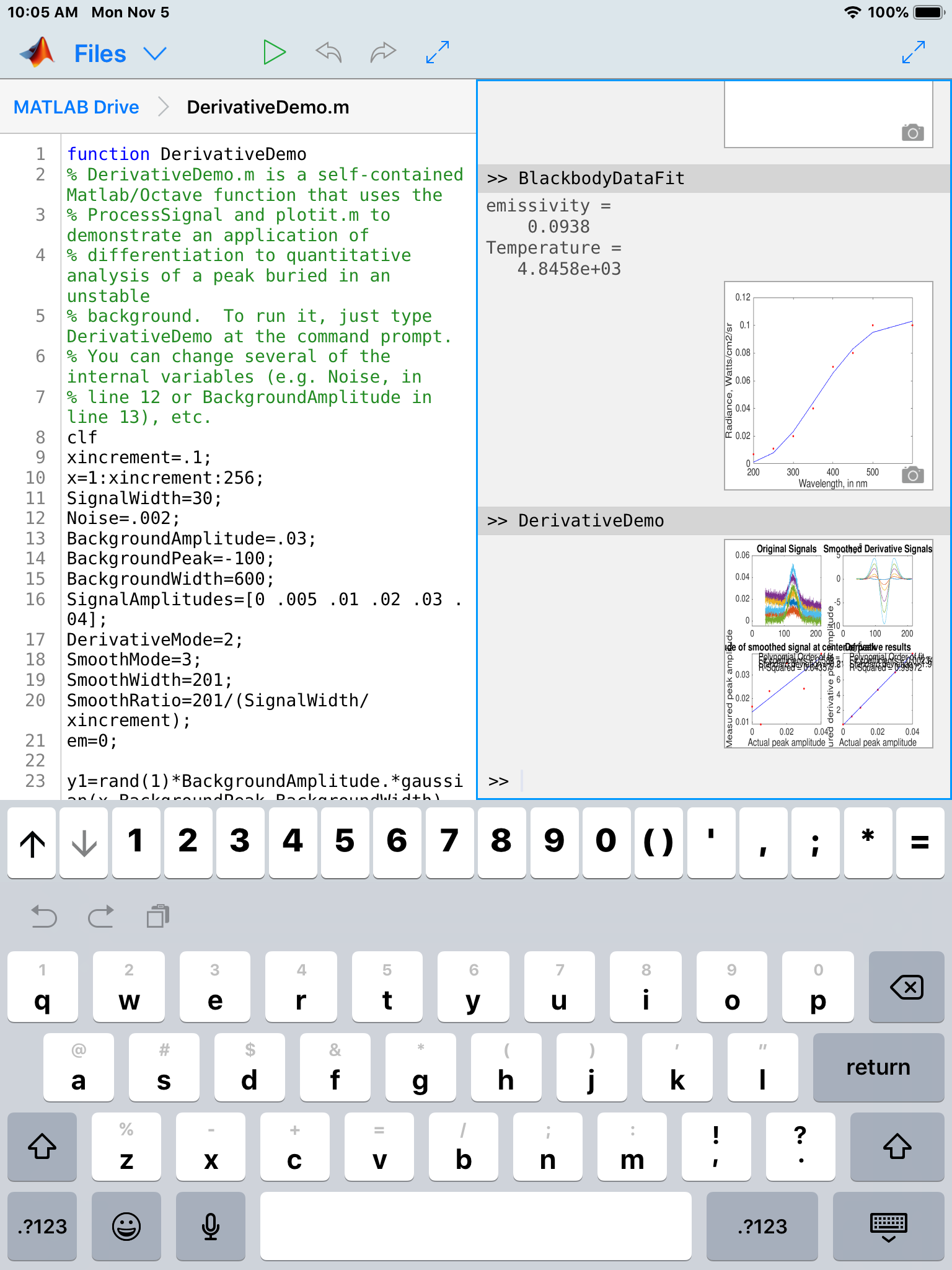version of Matlab is expensive (over \$2000) but don't let that frighten you - there are student and home versions that cost much less (as little as \$49 for a basic student version) and have all the capabilities to perform any of the methods detailed in this book at comparable execution speeds. There is also Matlab Online, which runs in a web browser (left); and the free Matlab Mobile app that runs Matlab on iPads and even iPhones (right). This requires only a basic student license and uses whatever functions, scripts, and data that you have previously uploaded to your free account on the Matlab cloud. Notably, all these versions have computational speeds within roughly a factor of 2 of each other, as shown by the text file TimeTrial.txt, which lists measured execution speeds for for a variety of signal processing tasks running on four different hardware/software configurations: Matlab 2020b, Matlab Online, R2018b, and Matlab Mobile (iPad).  See

GNU Octaveis a free alternative to Matlab that is "mostly compatible" . DspGURU says that Octave is "...a mature high-quality Matlab clone. It has the highest degree of Matlab compatibility of all the clones." Everything I said above about Matlab also works in Octave. In fact, the most recent versions of almost all of my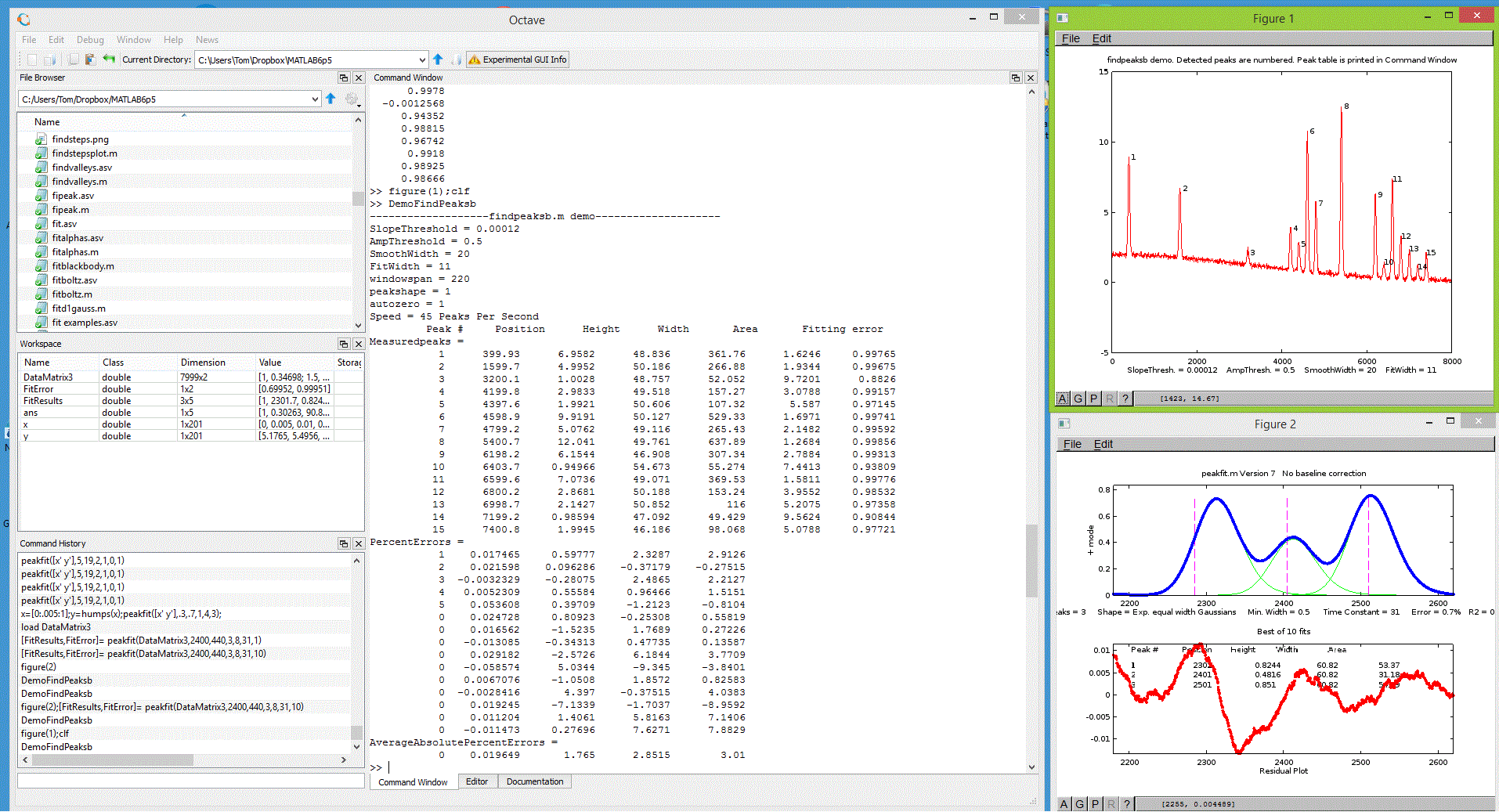Matlab functions, scripts, demos, and examples in this document will work in the latest version of Octave without change. (The keystroke-operated interactive functions requires separate Octave versions ipeakoctave.m, isignaloctave.m, and ipfoctave.m). If you plan to use Octave, make sure you get the current versions; many of them were updated for Octave compatibility in 2015 and this is an ongoing project. There is a FAQ that may help in porting Matlab programs to Octave. See Key Differences Between Octave & Matlab. There are Windows, Mac, and Unix versions of Octave; the Windows version can be downloaded from Octave Forge; be sure to install all the "packages". There is lots of help online: Google "GNU Octave" or see the YouTube videos for help. For signal processing applications specifically, Google "signal processing octave".  Note: the older Octave 3.6 can even run on a Raspberry Pi

Octave Version 6.4.0 has been released and is now available for download. It is much improved over older previous versions. New versions are introduced often. However, Octave is still computationally about 6 times slower on average than the latest Matlab version, depending on the task  (for some specific examples, see TimeTrial.txt). Bottom line: Matlab is better, but if you can't afford Matlab, Octave provides almost all of the functionality for 0% of the cost.

Python, a scripted language that is a free open-source alternative to Matlab, can be configured with almost all of the capabilities of Matlab for scientific signal processing. See https://terpconnect.umd.edu/~toh/spectrum/Python.html

Spreadsheetor Matlab/Octave/Python? For signal processing, Matlab/Octave and Python are faster and more powerful than using a spreadsheet, but it's safe to say that spreadsheets are more commonly installed on science workers' computers than Matlab or Python. For one thing, spreadsheets are easier to get started with and they offer flexible presentation and user interface design. Spreadsheets are better for data entry and are easily deployed on portable devices such as smartphones and tablets (e.g. using iCloud Numbers or the Excel app). Spreadsheets are concrete and more low-level, showing every single value explicitly in a cell. Matlab/Octave is more high level and abstract, because a single variable, punctuation, or function can do so much. An advantage of Matlab and Octave is that their function and script files ("m-files") are just plan text files with a ".m" extension, or ".py" in Python, so those files can be opened and inspected using any text editor, even on devices that do not have those programs installed. Also, user-defined functions can call other built-in or user-defined functions, which in turn can call other functions, and so on, allowing very complex high-level functions to be built up in layers. Fortunately, Matlab can easily read Excel .xls and .xlsx files and import the rows and columns into matrix variables.

Using the analogy of electronic circuits, spreadsheets are like old-school discrete component electronics, where every resistor, capacitor, and transistor is a discrete, macroscopic entity that you can see and manipulate directly. A function-based programming language like Matlab/Octave is more like micro-electronics, where the functions (the "m-files" that begin with "function...") are the integrated circuit "chips", that condense complex operations into one package with documented input and output pins (the function's input and output arguments) that you can connect to other functions, but which hide the internal details (unless you care to look at the code, which you always can do). A good example is the ubiquitous "555 timer", an 8-pin timer, pulse generator, and oscillator chip introduced in 1972, which has since become the most popular integrated circuit ever manufactured. These days, almost all electronics is done with chips of one type or another, because it's easier to understand the relatively small number of inputs and outputs of a chip than to deal with the greater number of internal components.

Much of Matlab/Octave is actually written in the Matlab/Octave language, using more basic functions to build more complex ones. You can write new functions of your own that essentially extend the language in whatever direction you need. Just remember to include your custom functions whenever you share you code with others.

The bottom line is that spreadsheets are easier at first, but in my experience, sooner or later, writing in Matlab/Octave/Python is more productive. This point is demonstrated by the comparison of both platforms multilinear regression in multicomponent spectroscopy (RegressionDemo.xls vs the Matlab/Octave CLS.m), and particularly by the dramatic difference between the spreadsheet and Matlab/Octave approaches to finding and measuring peaks in signals (i.e. a 250Kbyte spreadsheet vs a 7Kbyte script that's 50 times faster). If you have lots of data and you need to run it through a multi-step customized process automatically, hands-off, and as quickly as possible, then Matlab or Python is a great way to go. It's much easier to write a script that will automate the hands-off processing of volumes of data stored in separate data files on your computer; an example is given in Appendix X.

Both spreadsheets and scripted language programs have a huge advantage over commercial end-user programs and compiled freeware programs such as SPECTRUM; the scripts and functions can be inspected and modified by the user to customize the routines for specific needs. Simple changes are easy to make with little or little knowledge of programming. For example, you could easily change the labels, titles, colors, or line style of the graphs - in Matlab or Octave programs, search for "title(", "label(" or "plot(". My code often contains comments that indicate places where specific changes can easily be made: just use Find... to search for the word "change". You are invited to modify my scripts and functions as you wish.

Hint: This site contains many links to code and data examples, Matlab/Octave scripts, enlarged graphics, screen images, and spreadsheet templates; to view these alongside the text, right-click and select "Open link in new window".This page is part of "A Pragmatic Introduction to Signal Processing", created and maintained by Prof. Tom O'Haver , Department of Chemistry and Biochemistry, The University of Maryland at College Park. Comments, suggestions and questions should be directed to Prof. O'Haver at toh@umd.edu. Updated June, 2022.